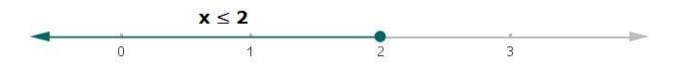# Solve each of the following in equations and represent the solution set on

Question:

Solve each of the following in equations and represent the solution set on the number line.

$\frac{(2 x-1)}{3} \geq \frac{(3 x-2)}{4}-\frac{(2-x)}{5}$ where $x \in \mathbf{R}$.

Solution:

Given:

$\frac{(2 x-1)}{3} \geq \frac{(3 x-2)}{4}-\frac{(2-x)}{5}$, where $x \in R$

Multiplying by 60 on both the sides in the above equation.

$(60) \frac{(2 x-1)}{3} \geq(60) \frac{(3 x-2)}{4}-(60) \frac{(2-x)}{5}$

$20(2 x-1) \geq 15(3 x-2)-12(2-x)$

$40 x-20 \geq 45 x-30-24+12 x$

$40 x-20 \geq 57 x-54$

Now, Adding 20 on both the sides in the above equation

$40 x-20+20 \geq 57 x-54+20$

$40 x \geq 57 x-34$

Now, subtracting 57x from both the sides in the above equation

$40 x-57 x \geq 57 x-34-57 x$

$-17 x \geq-34$

Multiplying by -1 on both sides in the above equation

$(-17 x)(-1) \geq(-34)(-1)$

$17 x \leq 34$

Now, divide by 17 on both sides in the above equation

$\frac{17 x}{17} \leq \frac{34}{17}$

Therefore,

$x \leq 2$